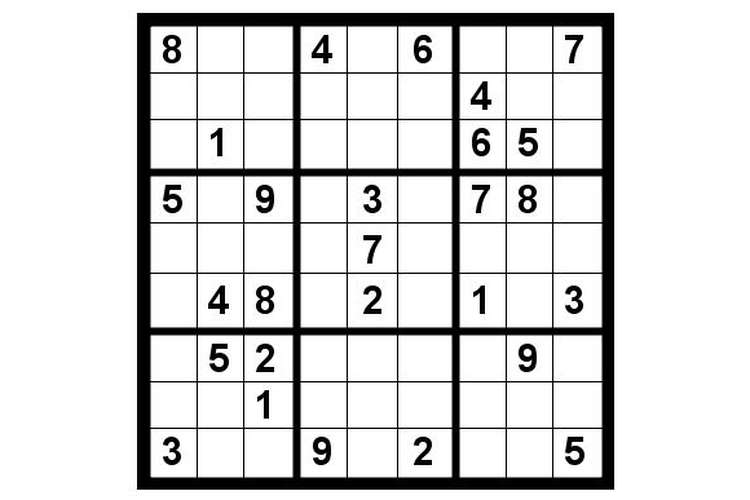# Computer Graphics Proof Reading Services## Introduction

Computer Graphics is chosen to have a clear understanding of software development life cycle and how the graphics are implemented. Computer graphics will help in understanding the intricacies of Java programming with Swing. Computer graphics thus made will help in the future to clearly understand what the project does and how it is implemented. This project can be thus taken in the future for further implementation and improvement.

### Rules

The game will be user-interactive game called Sudoku. The rule of the game are listed below

• Sudoku should follow all its standard rules.
• The number once entered in any horizontal line should not occur once again in the same line.
• The number once entered in any vertical line should not occur once again in the same line.
• The number once entered in any square matrix of 3*3 should not be repeated.
• User can move right, left, up and down in any fashion unless the cursor is on extreme right or left.
• The user will be provided with validation if the number entered is the correct number, as soon as the user leaves the cell after typing the number. The wrong numbers will be colored RED.

### Levels

The application will provide the user with Sudoku puzzle based on the user selected level of difficulty.  There will be three difficulty levels as follow -

• Easy -If the user selects an easy puzzle then the program will give a puzzle with lot of numbers given.
• Medium -If the user selects a medium puzzle the program will give a puzzle with less numbers than easy level.
• Hard -If the user selects hard difficulty then the program will give a puzzle with fewer numbers.

### Solution Analysis

The Sudoku puzzle is a NP complete problem as the grid contains n * n blocks. We cannot develop this problem by polynomial time algorithm and hence can only be solved by heuristic algorithm (Juillerat, 2012). To solve the Sudoku puzzle generally a heuristicalgorithm which follows a set of designed moves to achieve the desired result. Both basic and advanced information technology have to be used for solving this problem. For designing of this puzzle there are two types of logics which can be used which will make the program faster and efficient. The algorithms are named below:

The Brute Force Method: This method is used when all other techniques are failed and we have to make guesswork to complete the puzzle. This is used for very difficult of the Sudoku puzzles. In this method, we select the empty cell where least number of values is possible. Then out of those possible values one of the values is selected and then we try to complete the rest of the Sudoku with other numbers. If Sudoku is filled successfully then the puzzle is complete but if we are unable to complete the Sudoku or any of the basic rule get violated then we have to undo our first move and try with another possible number. We have to try this until the Sudoku is filled completely

The Backtracking Method: In this method, the Sudoku problem set is represented as a binary tree. The algorithm traverses this binary tree in a depth-first pattern to find a possible solution (Gurari, 1999). A partial solution set is maintained at every level of the tree. If on adding a new node to the partial set we get a new set which does not violate any rules of the game, then the new partial set is considered for the solution set. When at any level the solution the node violates any rule of the game, then the algorithm cannot go further deep in that chain of the tree. Then, we “back-track” to the previous consistent node and consider another branch originating from that node for our solution. If we encounter a scenario where all the branches of any node do not provide a solution set, then we consider that node to be inconsistent and move up or back-track for finding the next set of solutions. The primary advantage of this solution algorithm is that it is relatively faster than the other algorithms owing to the fact that it does not traverse each and every node of the tree to find a solution. Whenever it encounters an inconsistent node, it traverses back without considering any node lower to that inconsistent node in the chain. The game will also have online help for new users so that they can learn the game and learn the rules of the game.

### Modules

There will be two Modules –

• Game Selector
• Game Play
•  Architecture
• MVC (Model View Controller)
• Development Platform
• Java with Swing

### References

Nicolas Juillerat (2012) Sudoku Explainer. http://diuf.unifr.ch/people/juillera/Sudoku/Sudoku.html.
Gurari, Eitan (1999). Backtracking algorithms "CIS 680: DATA STRUCTURES: Chapter 19: Backtracking Algorithms